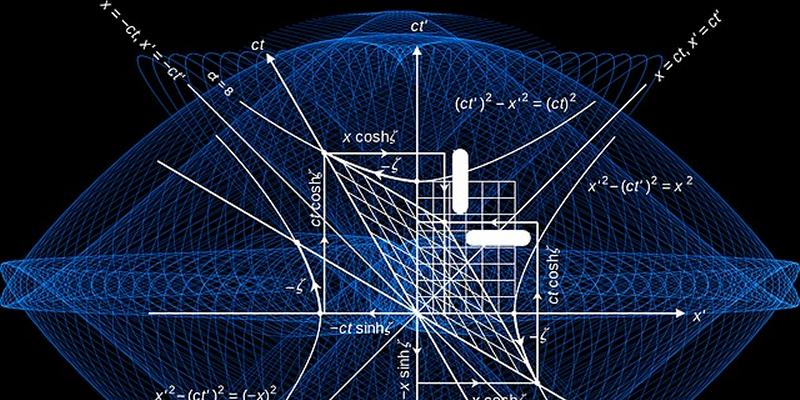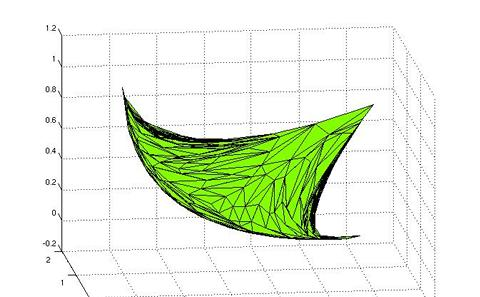The University of Southampton
Mathematical Sciences

# Research project: Numerical Methods for Nonlinear Optimisation

Currently Active:
Yes

The focus of our research is to develop numerical optimization methods for problems presenting multiple objectives and problems with a bilevel hierarchical structure, respectively. These problems have attracted a lot interest from the optimization continuity because of the mathematical challenges they present and the powerful framework they provide to model decision making problems with conflicting objectives or featuring two or more levels of decision making. We apply our research in these areas on space engineering, web science, transportation, network optimization, and game theory, as well as in methodological aspects of cutting plane generation.## Mathematical Programming for Data Mining

We are interested in data mining problems tackled from a mixed-integer (nonlinear) programming perspective. We mainly focus on three problems: hyperplane clustering, Euclidean norm classification, and piecewise affine regression. In the hyperplane clustering problem, we look for a collection of hyperplanes which best fit, in terms of Euclidean point-to-hyperplane distance, a given a set of points. The problem has applications to feature selection, image processing, and decision support in medical prognosis. We also tackle classification problems involving Euclidean point-to-hyperplane distances with spatial branch-and-bound techniques, also investigating approximation algorithms obtained by the adoption of different norms. Another relevant problem is that of fitting a piecewise affine function to data points subject to pairwise linear separability constraints, which we tackle via mixed-integer programing techniques, also involving symmetry breaking techniques.## Multiobjective Optimisation

In multicriteria (multiobjective) optimization, several objective functions have to be minimized simultaneously. Usually, no single point will minimize all given objective functions at once, and so the concept of optimality has to be replaced by the concept of Pareto-optimality or efficiency. A point is called Pareto-optimal or efficient, if there does not exist a different point with the same or smaller objective function values, such that there is a decrease in at least one objective function value. Two different efficient points will usually be not only quite different from each other in terms of objective function values, but also incomparable with each other: it will not be the case that one point is better than the other one with respect to all objective functions involved. Instead, the quality of the points will change across different objectives. Therefore, we have to gain as much information as possible about the solution set of a given problem, preferably by constructing a well-defined approximation to it.## Objects Reconstruction

The problem of recovering/reconstructing objects (e.g., images, digital signals) can be modelled by a discrete-type optimization problem. In the last decade, intensive research has led to recast this problem as a convex minimization problem with large chances to generate optimal solutions for the initial problem. In this area, I am interested in the problem of reconstructing phase information subject to some a priori information, with its application to obtain real space images of nanoscale structures by inverting coherent x-ray diffraction measurement data. The resulting optimization problem is non-convex because of the nature of the Fourier space projection onto the modulus set. The aim here is to develop optimization methods capturing the special structure of the problem while possibly guaranteeing the uniqueness of the reconstructed object.## Robust Optimisation

The project focuses on the formulation and solution of bilevel programming problems where the second level problem does not necessarily admit a (compact) set of optimality conditions. Among different applications of this growing area of research, we mention network routing problems with fairness constraints (for which a compact reformulation is possible) and leader-follower Stackelberg games (for which the lack of optimality conditions calls for specialized algorithms). From a methodological perspective, we are also interested in bilevel programming aspects of cutting plane generation. The attention is on bound-optimal cutting planes, a cutting plane paradigm which entails the solution of a bilevel cut generating problem with a linear program at the second level, and on the generation of rank inequalities for the maximum stable set problem, with extension to the generation of template-free inequalities for general integer programs.## Second Order Conic Optimisation (SOCP)

SOCPs are minimizations of real-valued functions over the intersection of a vector-valued map with a Cartesian product of ice cream cones. This problems have attracted a lot of interest in the last two decades, mainly because of the advantages that they provide in allowing an important number of non-linear and possibly non-convex problems to be converted into linear-type and convex programs. Secondly, in the mid-nineties, it was discovered that some powerful methods such as the interior point method can be extended from linear programs to SOCPs with a comparable level of efficiency. In this area, I am interested in the development of augmented Lagrangian-type solution methods for problems involving non-linear and possibly non-convex functions. I am also interested in connections that exist between SOCPs and bilevel programs and how they can help develop more efficient solution algorithms.## Spatial Branch-and-Bound for Nonlinear Programming

The project addresses the development of spatial branch-and-bound (sBB) methods for the solution of nonlinear programs with nonconvex constraints. The focus is on problems with one or many nonconvex 2-norm constraints stemming from data mining applications involving point-to-hyperplane Euclidean distances. We study approximation algorithms based on the use of polyhedral norms, and sBB methods where the introduction of (redundant) L1 and L1 norm constraints guarantees a faster improvement in the bound when branching. We also develop ad hoc branching techniques based on geometric approximations of the infeasible convex regions of such problems. Most of the work on bilevel programming also falls in this area, due to the nonlinearity which typically arises even when both 1st and 2nd level problems are linear.

### Related research groups

Operational Research
Computational Optimisation